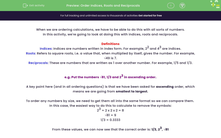# Order Indices, Roots and Reciprocals

In this worksheet, students will order a variety of numbers and expressions according to value, including indices, roots and reciprocals.Key stage:  KS 4

Year:  GCSE

GCSE Subjects:   Maths

GCSE Boards:   Pearson Edexcel, OCR, Eduqas, AQA,

Curriculum topic:   Number, Number Operations and Integers

Curriculum subtopic:   Structure and Calculation Combining Arithmetic Operations

Difficulty level:#### Worksheet Overview

When we are ordering calculations, we have to be able to do this with all sorts of numbers.

In this activity, we're going to look at doing this with indices, roots and reciprocals.

Definitions

Indices: Indices are numbers written in index form. For example, 22 and 43 are indices.

Roots: Refers to square roots, i.e. a value that, when multiplied by itself, gives the number. For example, √49 is 7.

Reciprocals: These are numbers that are written as 1 over another number. For example, 1/5 and 1/3.

e.g. Put the numbers √81, 1/3 and 23 in ascending order.

A key point here (and in all ordering questions) is that we have been asked for ascending order, which means we are going from smallest to largest.

To order any numbers by size, we need to get them all into the same format so we can compare them.

In this case, the easiest way to do this to calculate to remove the symbols:

23 = 2 x 2 x 2 = 8

√81 = 9

1/3 = 0.3333

From these values, we can now see that the correct order is: 1/3, 23, √81

In this activity, you will order a variety of numbers and expressions according to value, including indices, roots and reciprocals.

### What is EdPlace?

We're your National Curriculum aligned online education content provider helping each child succeed in English, maths and science from year 1 to GCSE. With an EdPlace account you’ll be able to track and measure progress, helping each child achieve their best. We build confidence and attainment by personalising each child’s learning at a level that suits them.

Get started